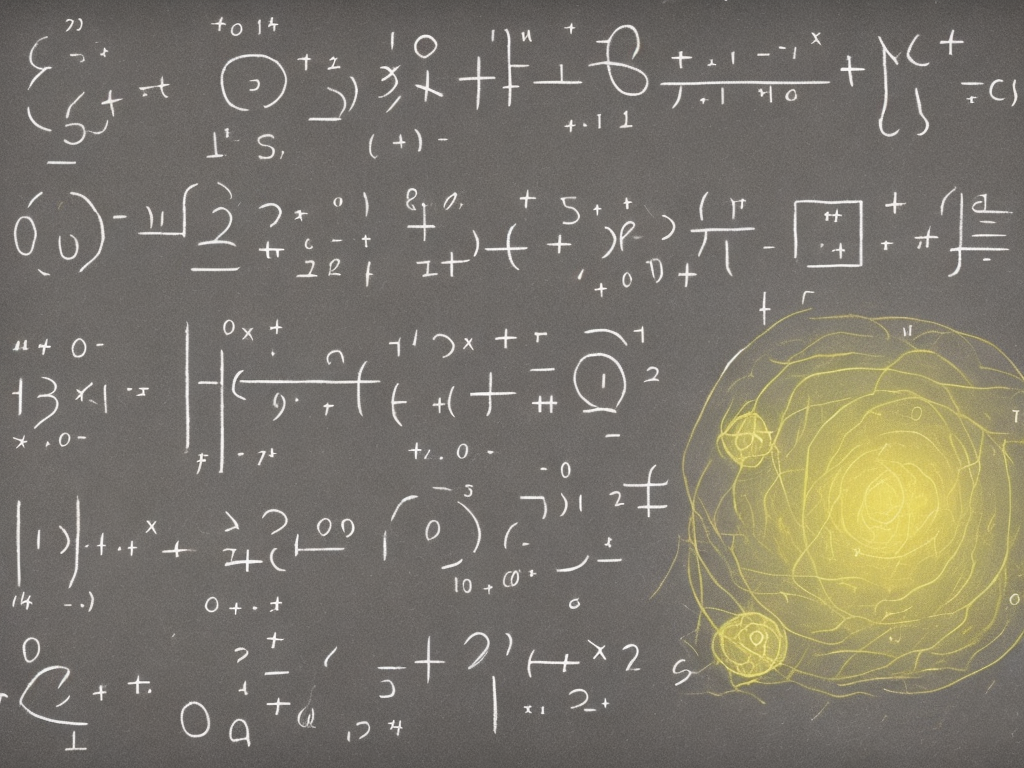tl;dr
To add fractions, find a common denominator by finding the least common multiple of the denominators, add the numerators and simplify the fraction (if necessary) by finding the greatest common factor of the numerator and denominator and dividing both by that number.Adding fractions can be tricky, but with a few simple steps, you can become a master at it in no time! In this article, we will explain how to add fractions step by step.

Step 1: Find a common denominator

Before we can add fractions, we need to make sure they have a common denominator. A denominator is the bottom number of a fraction and represents the total number of equal parts that make up a whole. To find a common denominator, we need to find the least common multiple (LCM) of the denominators of the fractions we want to add.

For example, let's say we want to add 1/3 and 1/4. The LCM of 3 and 4 is 12, so we need to convert both fractions so they have a denominator of 12. To do this, we need to multiply the numerator and denominator of each fraction by a number that will give us a denominator of 12. For the first fraction, we can multiply both the numerator and denominator by 4, since 3 multiplied by 4 is 12. This gives us 4/12. For the second fraction, we can multiply both the numerator and denominator by 3, since 4 multiplied by 3 is 12. This gives us 3/12.

Now that we have a common denominator, we can add the numerators of the fractions. The numerator is the top number of a fraction and represents the number of equal parts we are adding together. To add the numerators, we simply add them together and keep the denominator the same.

Using our example above, we can now add the two fractions: 4/12 + 3/12 = 7/12. So, the answer to 1/3 + 1/4 is 7/12.

Step 3: Simplify the fraction (if necessary)

If the fraction we get as an answer can be simplified, we should simplify it. Simplifying a fraction means dividing both the numerator and denominator by the same number to make the fraction smaller. To simplify a fraction, we need to find the greatest common factor (GCF) of the numerator and denominator and then divide both by that number.

For example, in our answer above of 7/12, both the numerator and denominator can be divided by 7. The greatest common factor of 7 and 12 is 1, so we can divide both by 7 to simplify the fraction. This gives us 1/12 as the simplified answer.

Let's look at another example. Say we want to add 2/5 and 1/10. To find a common denominator, we need to find the least common multiple of 5 and 10, which is 10. We can convert the first fraction to a denominator of 10 by multiplying the numerator and denominator by 2. This gives us 4/10. The second fraction already has a denominator of 10, so we can leave it as is. Now we can add the two fractions: 4/10 + 1/10 = 5/10. This fraction can be simplified since both the numerator and denominator can be divided by 5. This gives us a simplified answer of 1/2.